# RS Aggarwal Solutions for Class 9 Chapter 4: Linear Equations in Two Variables Exercise 4A

## RS Aggarwal Solutions for Class 9 Maths Exercise 4A PDF

In order to excel in the CBSE exam for Class 9, students can use the solutions of Exercise 4A available on the website. By practising regularly the students can obtain worthy results in the Class 9 exams by using the RS Aggarwal Solutions. The PDF of solutions of exercise 4A mainly helps students from the exam point of view. This exercise being the first in the fourth chapter mainly contains solved problems based on the concept of Linear Equations in Two Variables. RS Aggarwal Solutions for Class 9 Maths Chapter 4 Linear Equations in Two Variables Exercise 4A are provided here.

## RS Aggarwal Solutions for Class 9 Chapter 4: Linear Equations in Two Variables Exercise 4A Download PDF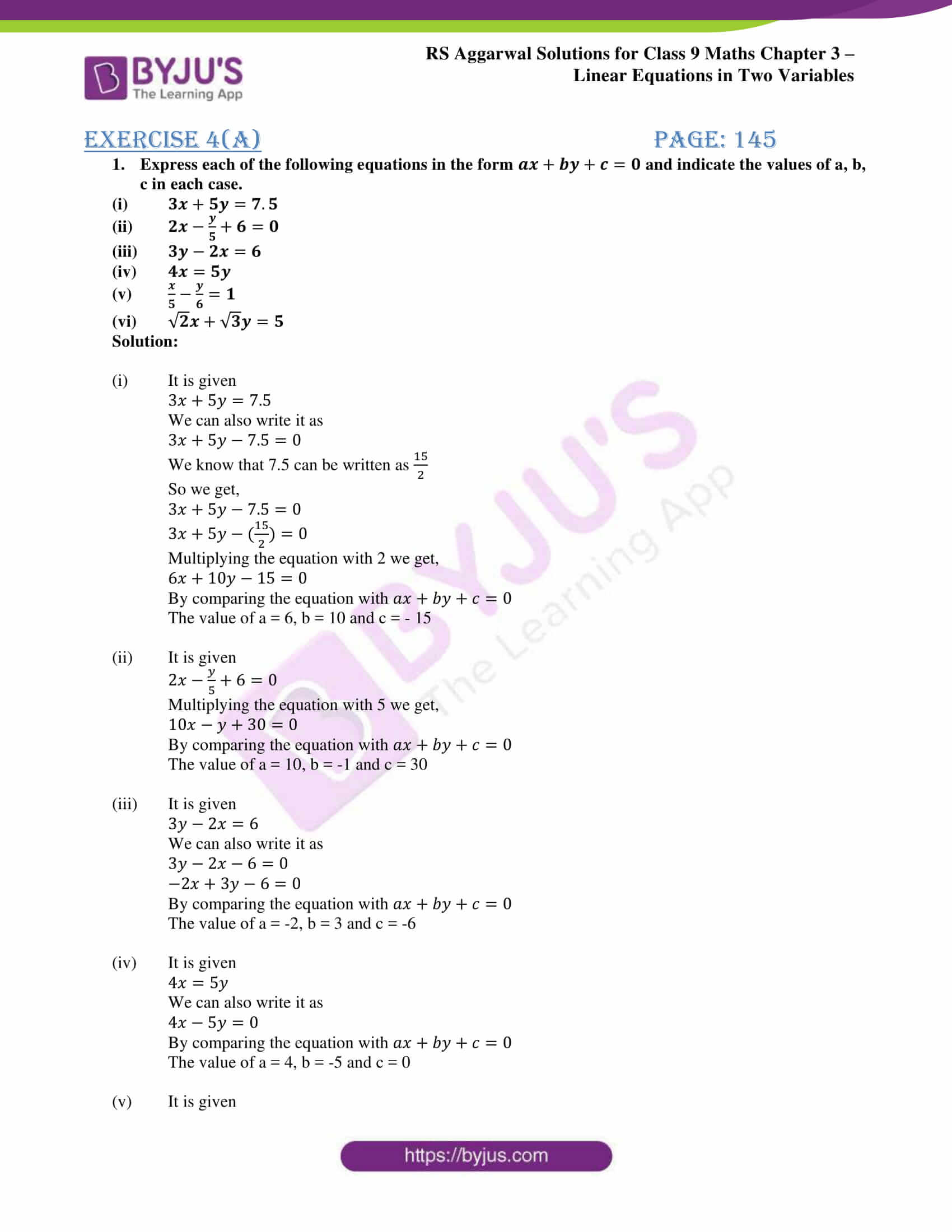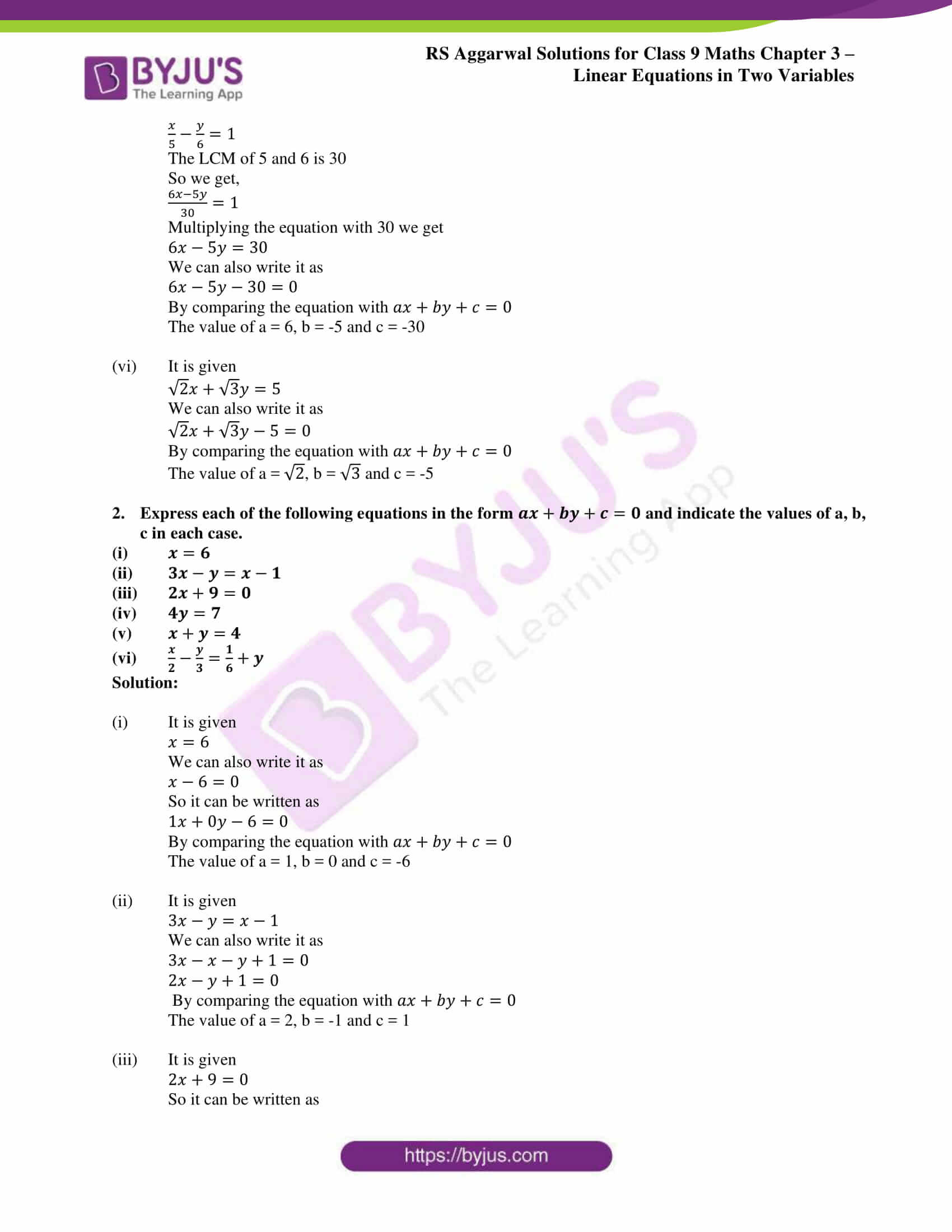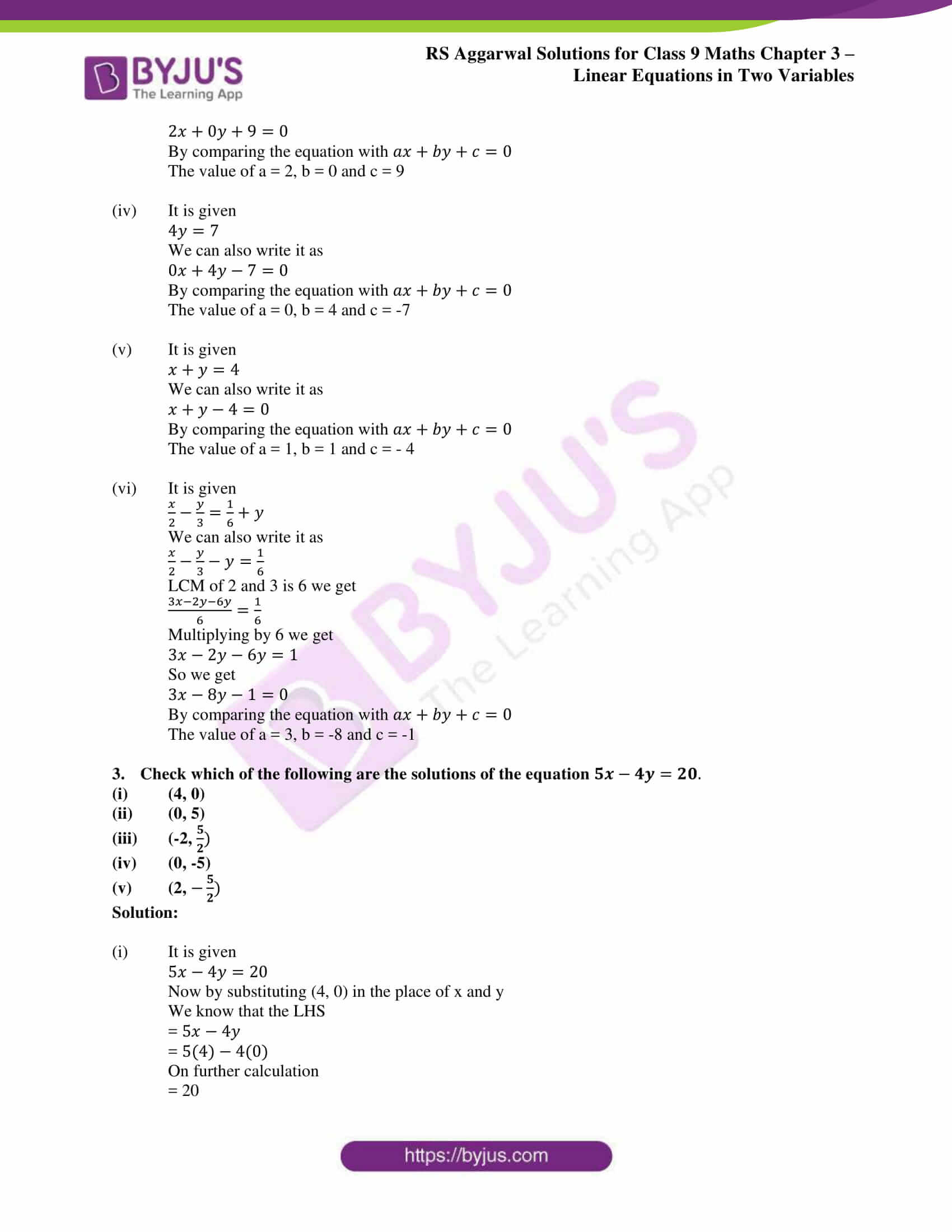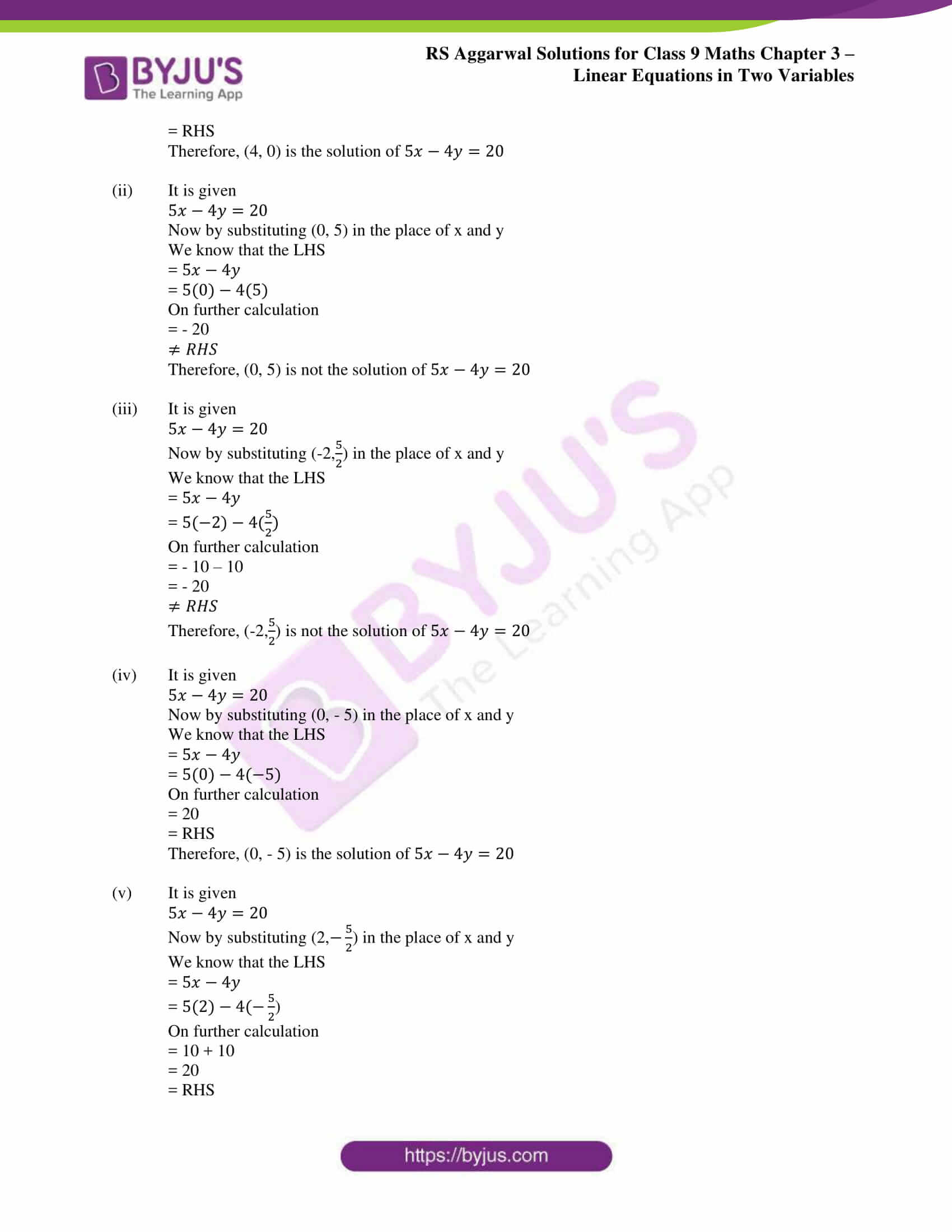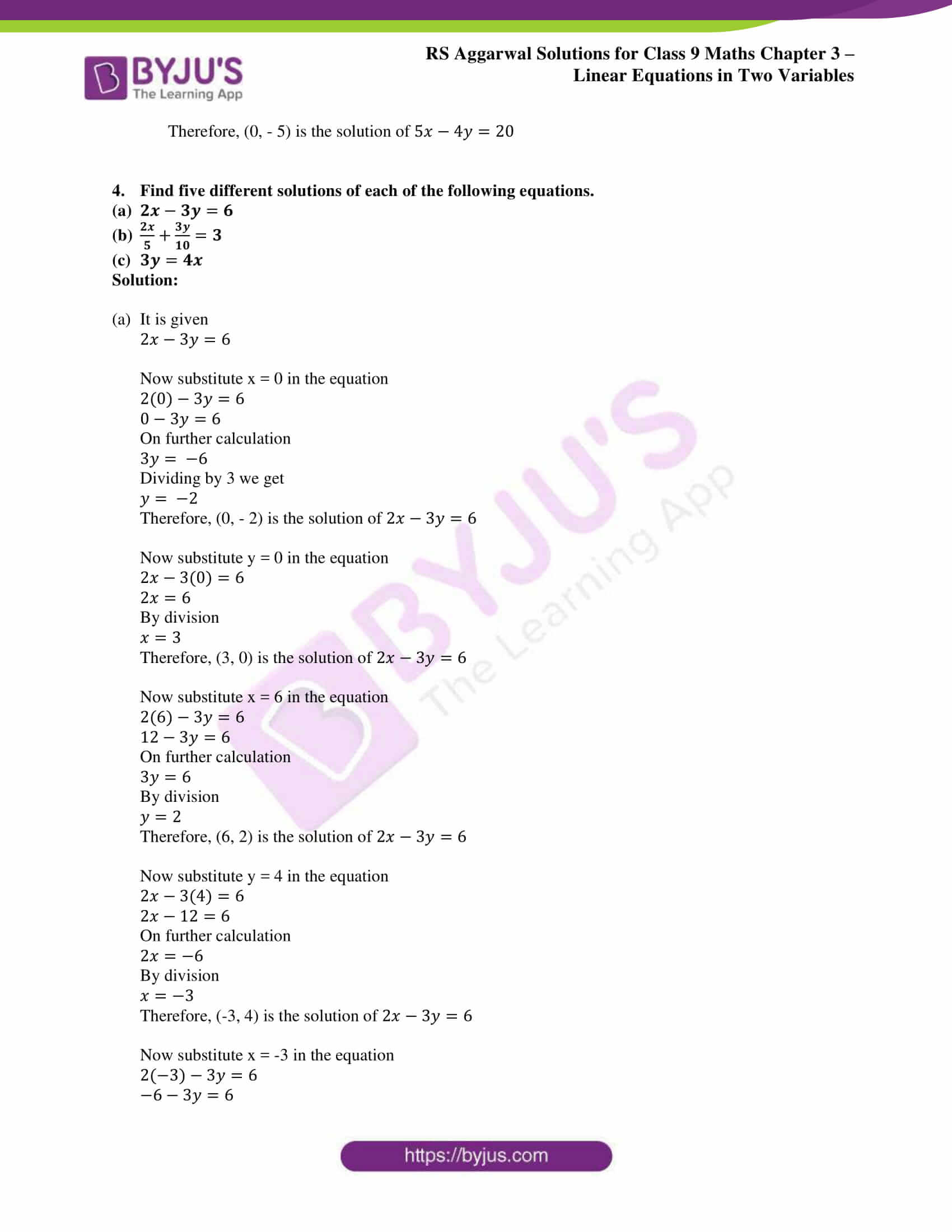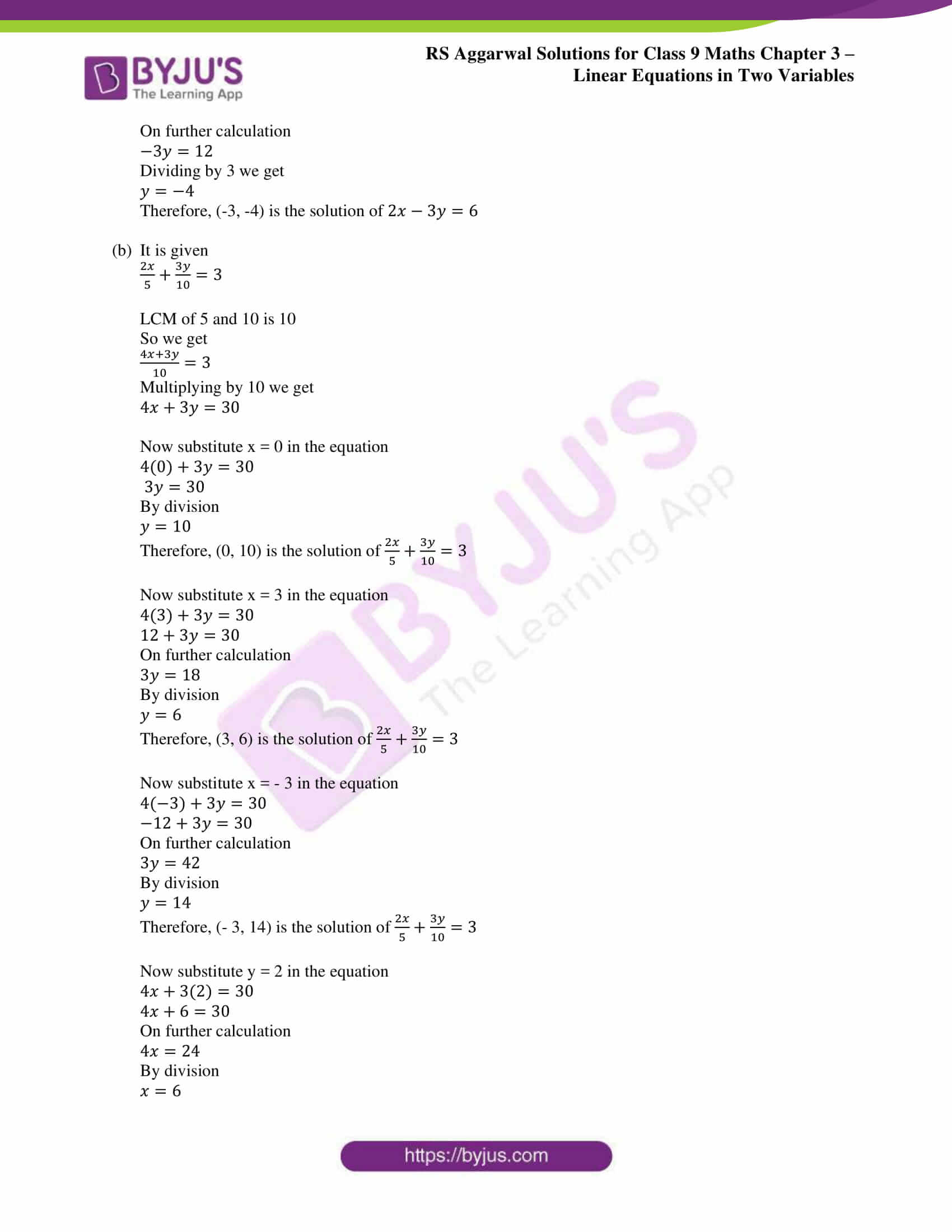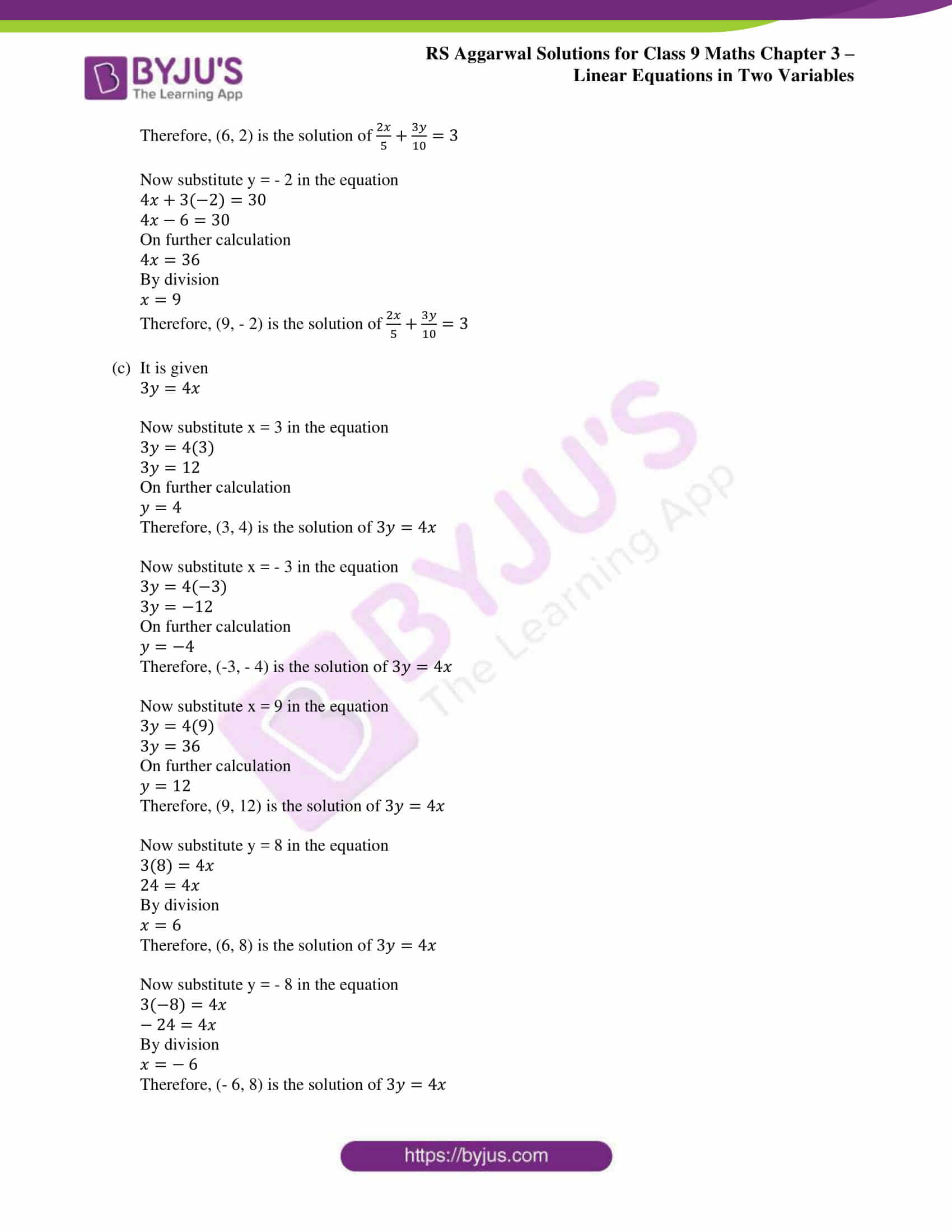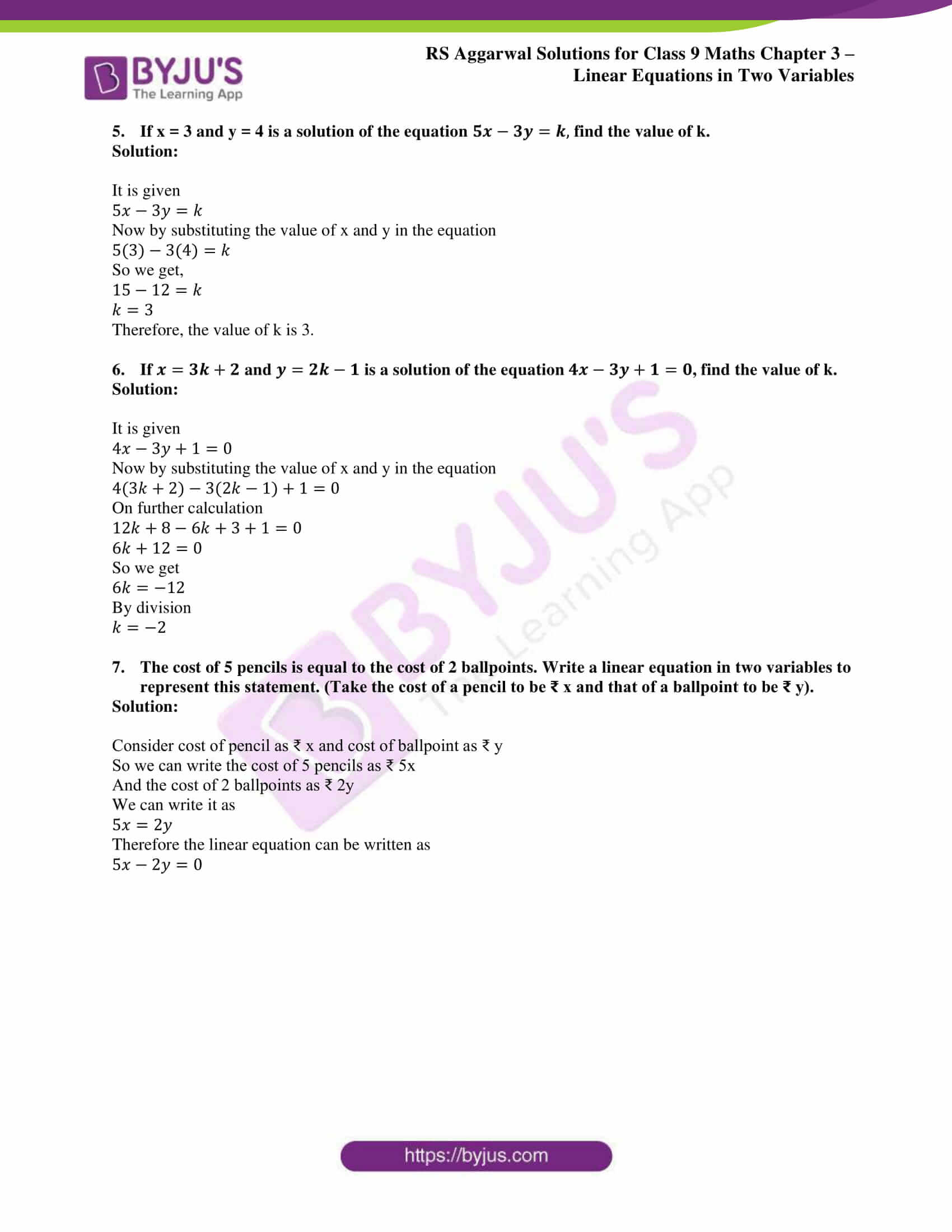### Access other exercise solutions of class 9 Maths Chapter 4: Linear Equations in Two Variables

Exercise 4B Solutions 12 Questions

### RS Aggarwal Solutions Class 9 Maths Chapter 4 – Linear Equations in Two Variables Exercise 4A

RS Aggarwal Solutions Class 9 Maths Chapter 4 Linear Equations in Two Variables Exercise 4A is the first exercise of the fourth chapter containing solutions based on Linear Equations in Two Variables.

### Key features of RS Aggarwal Solutions for Class 9 Maths Chapter 4: Linear Equations in Two Variables Exercise 4A

• The RS Aggarwal Solutions are solved in a precise manner easily understandable by the students to clear exams.
• The main aim of our subject experts is to provide better clarity about the concepts among the students.
• The students on a regular practice of exercise wise problems can obtain a strong foothold in a subject like Mathematics.
• Very lengthy questions which require many steps can be easily remembered by the students by using RS Aggarwal Solutions.